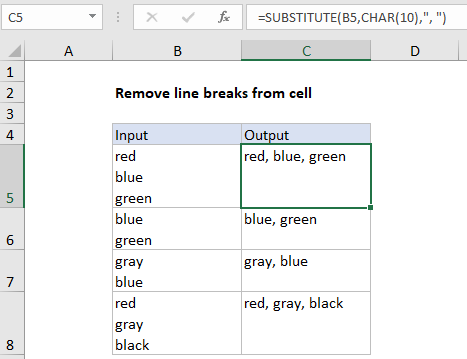Remove line breaks in a cell in Excel

To remove line breaks from a cell, or from text inside a formula, you can use a formula based on the SUBSTITUTE and CHAR functions.

Formula

=SUBSTITUTE(A1,CHAR(10),", ")Explanation

In the example shown, the formula in C5 is:

=SUBSTITUTE(B5,CHAR(10),", ")

which replaces line breaks in B5 with commas.

How this formula works

First, you should know that Excel contains two functions, CLEAN and TRIM, that can automatically remove line breaks and extra spaces from text. For example to strip all line breaks from a cell, you could use:

=CLEAN(B5)

In this case, however, we are removing line breaks and replacing them with commas, so we are using the SUBSTITUTE function instead of CLEAN. SUBSTITUTE can locate matching text anywhere in a cell, and replace it with the text of your choice. SUBSTITUTE can accept up to four arguments, but we are using only the first three like this:

=SUBSTITUTE(B5,CHAR(10),", ")

The text comes from cell B5.

Also See:   How to count total words in a range in Excel

The “old text” is entered as CHAR(10). This will match the line break character used in Windows.

The “new text” is entered as “, “. This translates to a comma plus one space. We need the quotes because this is a text value.

SUBSTITUTE then replaces all line breaks in the cell with commas and returns the final result as text in C5. Because “old text” is an argument, you can change the comma to any other text you like.

Also See:   How to calculate Number of Instances in Excel